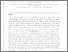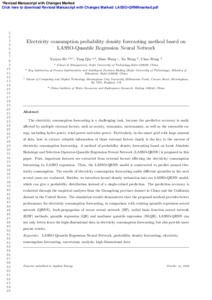# Electricity consumption probability density forecasting method based on LASSO-Quantile Regression Neural Network

He, Yaoyao and Qin, Yang and Wang, Shuo and Wang, Xu and Wang, Chao (2018) Electricity consumption probability density forecasting method based on LASSO-Quantile Regression Neural Network. Applied Energy, 233. pp. 565-575.Preview
Text
APEN-D-18-04592R3-pages-6-31.pdf - Accepted Version

## Abstract

The electricity consumption forecasting is a challenging task, because the predictive accuracy is easily affected by multiple external factors, such as society, economics, environment, as well as the renewable energy, including hydro power, wind power and solar power. Particularly, in the smart grid with large amount of data, how to extract valuable information of those external factors timely is the key to the success of electricity consumption forecasting. A method of probability density forecasting based on Least Absolute Shrinkage and Selection Operator-Quantile Regression Neural Network (LASSO-QRNN) is proposed in this paper. First, important features are extracted from external factors affecting the electricity consumption forecasting by LASSO regression. Then, the LASSO-QRNN model is constructed to predict annual electricity consumption. The results of electricity consumption forecasting under different quantiles in the next several years are evaluated. Besides, we introduce kernel density estimation into our LASSO-QRNN model, which can give a probability distribution instead of a single-valued prediction. The prediction accuracy is evaluated through the empirical analyses from the Guangdong province dataset in China and the California dataset in the United States. The simulation results demonstrate that the proposed method provides better performance for electricity consumption forecasting, in comparison with existing quantile regression neural network (QRNN), back-propagation of errors neural network (BP), radial basis function neural network (RBF), quantile regression (QR) and nonlinear quantile regression (NLQR). LASSO-QRNN can not only better learn the high-dimensional data in electricity consumption forecasting, but also provide more precise results.

Item Type: Article 20 October 2018 G400 Computer ScienceG700 Artificial Intelligence Faculty of Computing, Engineering and the Built Environment > School of Computing and Digital Technology Shuo Wang 20 Nov 2018 15:23 20 Nov 2018 15:23 http://www.open-access.bcu.ac.uk/id/eprint/6525View Item